Olympiad Test: Motion & Measurement of Distances

# Olympiad Test: Motion & Measurement of Distances

Test Description

## 20 Questions MCQ Test Science Class 6 | Olympiad Test: Motion & Measurement of Distances

Olympiad Test: Motion & Measurement of Distances for Class 6 2022 is part of Science Class 6 preparation. The Olympiad Test: Motion & Measurement of Distances questions and answers have been prepared according to the Class 6 exam syllabus.The Olympiad Test: Motion & Measurement of Distances MCQs are made for Class 6 2022 Exam. Find important definitions, questions, notes, meanings, examples, exercises, MCQs and online tests for Olympiad Test: Motion & Measurement of Distances below.
Solutions of Olympiad Test: Motion & Measurement of Distances questions in English are available as part of our Science Class 6 for Class 6 & Olympiad Test: Motion & Measurement of Distances solutions in Hindi for Science Class 6 course. Download more important topics, notes, lectures and mock test series for Class 6 Exam by signing up for free. Attempt Olympiad Test: Motion & Measurement of Distances | 20 questions in 20 minutes | Mock test for Class 6 preparation | Free important questions MCQ to study Science Class 6 for Class 6 Exam | Download free PDF with solutions
 1 Crore+ students have signed up on EduRev. Have you?
Olympiad Test: Motion & Measurement of Distances - Question 1

### A cyclist moves from a certain point P and goes round a circle of radius a and reaches Q, exactly at the other side of the point P as shown in figure. The displacement of the cyclist would be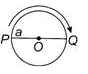Detailed Solution for Olympiad Test: Motion & Measurement of Distances - Question 1

Displacement of the cyclist = Shortest distance between P and Q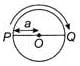= PO+OQ = a+a = 2a

Olympiad Test: Motion & Measurement of Distances - Question 2

### Read the given statements and select the correct option, Statement 1: The displacement of a body may be zero, though distance can be finite. Statement 2: If a body moves such that finally it arrives at initial point, then displacement is zero while distance is finite.

Detailed Solution for Olympiad Test: Motion & Measurement of Distances - Question 2

When a body moves from one position to another, the shortest distance measured between the initial and the final positions of the moving body in a particular direction is called displacement. The actual length of the path travelled by a moving body in a given interval of time is called the distance travelled by that body. So displacement of a body may be zero, though distance can be finite. For example, in case of circular motion if the body comes back to its initial position then distance travelled is finite but displacement is zero.

Olympiad Test: Motion & Measurement of Distances - Question 3

### In circular motion, the

Detailed Solution for Olympiad Test: Motion & Measurement of Distances - Question 3

When an object moves along a circular path, it is called circular motion. During such a motion, the direction of motion at any point is given by the tangent at that point which changes continuously.

Olympiad Test: Motion & Measurement of Distances - Question 4

What causes a moving body to resist a change in its state of motion?

Detailed Solution for Olympiad Test: Motion & Measurement of Distances - Question 4

The property of a body by virtue of which it resists any change in its state of rest or of motion in a straight line on its own is called its inertia.

Olympiad Test: Motion & Measurement of Distances - Question 5

A rider on horse falls back when horse starts running all of a sudden because

Detailed Solution for Olympiad Test: Motion & Measurement of Distances - Question 5

A rider on horse falls back when horse starts running, all of a sudden because the rider is in state of rest initially and later inertia of rest keeps the upper part of body at rest while lower part of the body moves forward with horse.

Olympiad Test: Motion & Measurement of Distances - Question 6

One centimeter on a scale is divided into 20 equal divisions. The least count (minimum value) of this scale is

Detailed Solution for Olympiad Test: Motion & Measurement of Distances - Question 6

As 20 divisions = 1 cm
∴ 1 division = 1/20 cm
= 0.05cm
= 0.5 mm
Hence, least count of this scale = 0.5 mm

Olympiad Test: Motion & Measurement of Distances - Question 7

Match the following and select the correct option from the codes given below.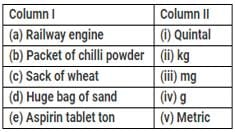Olympiad Test: Motion & Measurement of Distances - Question 8

Which of the following motions is/are periodic as well as oscillatory motion?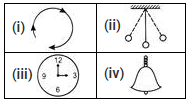Detailed Solution for Olympiad Test: Motion & Measurement of Distances - Question 8

Only (ii) shows the periodic as well as oscillatory motion. It is showing to and fro as well as motion which is repeating after regular time interval.

Olympiad Test: Motion & Measurement of Distances - Question 9

Which of the following statements is correct?

Detailed Solution for Olympiad Test: Motion & Measurement of Distances - Question 9

Motion of soldiers on march past is rectilinear motion; Every oscillatory motion is also a periodic motion; Hockey player running after a ball is random motion.

Olympiad Test: Motion & Measurement of Distances - Question 10

Four pieces of wooden sticks P, Q, R and S are placed along the length of 15 cm long scale as shown in figure. What is the average length of these sticks?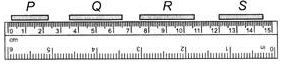Detailed Solution for Olympiad Test: Motion & Measurement of Distances - Question 10

P = 2.1 cm; Q = 3.0 cm; R = 3.1 cm; S = 2.5 cm
Average length of these sticks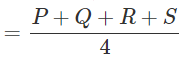= 2.6cm

Olympiad Test: Motion & Measurement of Distances - Question 11

Direction: Observe the given situation and answer the following question.
Rahul and Ravi are playing in a ground. They start running from the same point X simultaneously in the ground and reach point Y at the same time by following paths marked 1 and 2 respectively, as shown in the figure.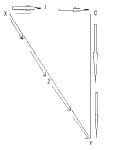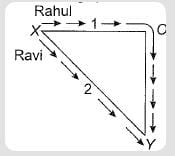Q. Which one of the following paths does show the displacement?

Detailed Solution for Olympiad Test: Motion & Measurement of Distances - Question 11

Rahul takes path XOY and Ravi takes path XY. Displacement is minimum distance between initial and final point = XY.

Olympiad Test: Motion & Measurement of Distances - Question 12

Rahul and Ravi are playing in a ground. They start running from the same point X simultaneously in the ground and reach point Y at the same time by following paths marked 1 and 2 respectively, as shown in the figure.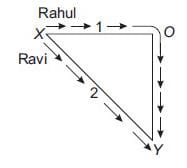Q. Which of the following is correct statement for the given situation?

Detailed Solution for Olympiad Test: Motion & Measurement of Distances - Question 12

Rahul and Ravi both cover different distances XOY and XY respectively at the same time. Rahul covers more distance than Ravi in the same time. Hence Rahul covers longer distance with a higher speed.

Olympiad Test: Motion & Measurement of Distances - Question 13

Which of the following statements is incorrect?

Detailed Solution for Olympiad Test: Motion & Measurement of Distances - Question 13

Rectilinear motion is another name for straight-line motion.

Olympiad Test: Motion & Measurement of Distances - Question 14

While measuring the length of a wooden box, the reading at one end is 1.5 cm and the other end is 4.7 cm. What is the length of the wooden box?

Detailed Solution for Olympiad Test: Motion & Measurement of Distances - Question 14

The length of the wooden box = Difference between the reading at both the ends = 4.7−1.5 = 3.2 cm

Olympiad Test: Motion & Measurement of Distances - Question 15

Motion of strings of a guitar shows
(i) oscillatory motion
(ii) vibratory motion
(iii) random motion
(iv) rotational motion

Detailed Solution for Olympiad Test: Motion & Measurement of Distances - Question 15

Motion of strings of a guitar shows oscillatory as well as vibratory motion.

Olympiad Test: Motion & Measurement of Distances - Question 16

Study the given Venn diagram, Which of the motions described by different bodies are most likely to be I, II and III?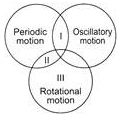Detailed Solution for Olympiad Test: Motion & Measurement of Distances - Question 16

Option 1,2 and 3 are rotatory motion. Rotatory motion, also referred to as rotational motion or circular motion, is physical motion that happens when an object rotates or spins on an axis.

Olympiad Test: Motion & Measurement of Distances - Question 17

Two identical metal balls A and 8 moving in opposite directions with different speeds hit each other at point X as shown in the figure. Changes will most likely appear in their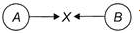1. Shapes
2. Speeds
3. Directions
4. Volumes

Detailed Solution for Olympiad Test: Motion & Measurement of Distances - Question 17

In case of collision, changes are most likely to be appear in the speed and direction of both the balls.

Olympiad Test: Motion & Measurement of Distances - Question 18

A pendulum swings backwards and forwards passing through Y, middle point of the oscillation. The first time the pendulum passes through Y, a stopwatch is started.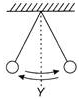The twenty-first time the pendulum pass through Y, the stopwatch is stopped. The reading is T. What is the time period of the pendulum?

Detailed Solution for Olympiad Test: Motion & Measurement of Distances - Question 18

The time taken to complete one oscillation is called the time period of a pendulum.Here the time period is the time taken to move from Y to B then back to A and then to Y. The reading of stopwatch = T Number of oscillations = 10

∴  Time taken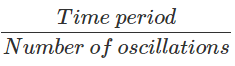= T/10

Olympiad Test: Motion & Measurement of Distances - Question 19

Given figure shows a measuring cylinder (incm3) before and after the immersion of an irregular solid object. The volume of the object is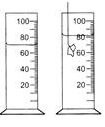Detailed Solution for Olympiad Test: Motion & Measurement of Distances - Question 19

Initial volume of water in the cylinder, (before the immersion of the object)
V= 70cm3
Final volume in the cylinder (after the immersion of the object)
V= 82cm3
Hence, volume of the object immersed in the cylinder = Final volume - Initial volume
= 82 cm3−70 cm
= 12 cm3

Olympiad Test: Motion & Measurement of Distances - Question 20

A piece of thread folded 6 times is placed along a 15 cm long measuring scale as shown in the figure. The length of the thread is between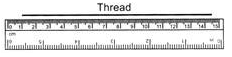Detailed Solution for Olympiad Test: Motion & Measurement of Distances - Question 20

The length of the thread is between 0.50m-1.0m

## Science Class 6

113 videos|173 docs|48 tests
 Use Code STAYHOME200 and get INR 200 additional OFF Use Coupon Code
Information about Olympiad Test: Motion & Measurement of Distances Page
In this test you can find the Exam questions for Olympiad Test: Motion & Measurement of Distances solved & explained in the simplest way possible. Besides giving Questions and answers for Olympiad Test: Motion & Measurement of Distances, EduRev gives you an ample number of Online tests for practice

## Science Class 6

113 videos|173 docs|48 tests